# Plot a graph.### Precalculus: Mathematics for Calcu...

6th Edition
Stewart + 5 others
Publisher: Cengage Learning
ISBN: 9780840068071### Precalculus: Mathematics for Calcu...

6th Edition
Stewart + 5 others
Publisher: Cengage Learning
ISBN: 9780840068071

#### Solutions

Chapter 1.9, Problem 77E
To determine

## Plot a graph.

Expert Solution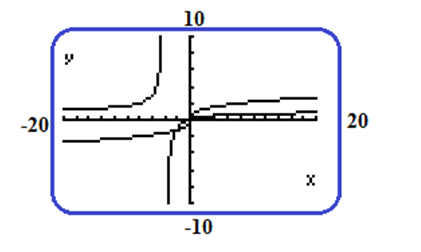### Explanation of Solution

Calculation:

Consider the equations,

y=x1/3 And  y=xx+4

The information is entered by student in the equation editor of the calculator as

y=x1/3  and y=x/x+4

The display is as follows: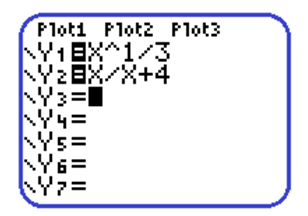Set Xmin=20,Ymin=10,and,Ymax=10.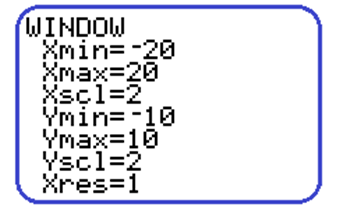The graph of the function are given below: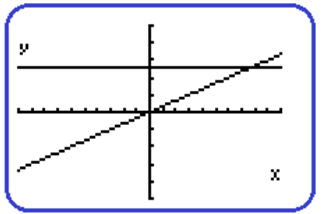The graphs displayed are different from the graphs the student wanted. The wrong graphs are displayed because the student forgot to put parentheses at appropriate places.

The information shown below in the equation editor of the calculator.

y=x(1/3)  and y=x/(x+4)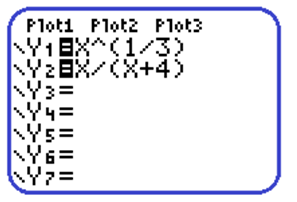The correct graphs are as follows:### Have a homework question?

Subscribe to bartleby learn! Ask subject matter experts 30 homework questions each month. Plus, you’ll have access to millions of step-by-step textbook answers!